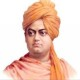# Quantitative Aptitude: Data Interpretation Questions Set 54

Quantitative Aptitude Questions for IBPS RRB/PO/Clerk, SBI PO, NIACL, NICL, RBI Grade B/Assistant, BOI, Bank of Baroda and other competitive exams.

Directions(1-5): Study the following table and carefully answer the following questions.Note:
I). Net Revenue = Gross Revenue – Amount Allocated for commission – Amount allocated for discount and others
II). Few values are missing in the table. A candidate is expected to calculate the missing value , if it is required to answer the given question, on the basis of the given data and the information.

1. Amount allocated for commission in March is what percent less than Amount allocated for commission in July?
A) 22%
B) 40%
C) 35%
D) 32%
E) 28%
Option A
Solution:
Amount allocated for commission:
March = Rs.31200
July = Rs. 40000
Required % = [(40000 – 31200)/40000]*100 = 22%
2. What is the difference between Net Revenue of the Magazine in April and its Gross Revenue in June?
A) Rs.115000
B) Rs.150000
C) Rs.126000
D) Rs.185500
E) Rs.117200
Option C
Solution:
Net Revenue received in April = Rs.(320000 – 28000 – 16000)= Rs.27600
Gross revenue received in June = Rs.(330000 + 30000 + 42000) = Rs.402000
Required difference = 402000 – 276000 = Rs.126000
3. In July, if 40% of the Gross Revenue of the Magazine was collected from Advertisement, what was the amount of gross revenue collected form advertisement in that particular month?
A) Rs.115020
B) Rs.147850
C) Rs.24480
D) Rs.172000
E) Rs.114570
Option D
Solution:
Gross Revenue received in July = Rs.(362000 + 28000 + 40000)
= Rs.430000
= Rs. 172000
4. In May, the respective ratio of amount allocated for commission and amount allocated for discount and others was 4 : 3. What was the Gross Revenue of the Magazine in May?
A) Rs.152400
B) Rs.420000
C) Rs.458500
D) Rs.558800
E) Rs.452250
Option B
Solution:
Amount allocated for commission = 36000 * (4/3) = Rs.48000
Gross Revenue received in May =Rs.(336000 + 36000 + 48000)
=Rs.420000
5. In March, if Net Revenue of the Magazine was 85% of its Gross Revenue, what was the amount allocated for discount and others?
A) Rs.18500
B) Rs.25000
C) Rs.22800
D) Rs.17420
E) Rs.181500
Option C
Solution:
Net Revenue received in March = 360000 *(85/100) = Rs.306000
Amount allocated for discount and others = Rs. (360000 – 306000 – 31200) =Rs. 22800

Directions(6-10): Study the following graph carefully to answer the following questions:

Cost of three different fruits (in Rs. per kg) in five different cities1. What total amount will Mohan pay to the shopkeeper for purchasing 3 kg of Mangoes and 2 kg of pomegranate in Delhi?
A) Rs.480
B) Rs.500
C) Rs.630
D) Rs. 570
E) Rs.610
Option D
Solution:
Amount paid by Mohan = (3 * 130 + 2 * 90) = Rs. 570
2. What is the respective ratio between the cost of one kg of mangoes from Chennai and the cost of one kg of coconut from Mumbai?
A) 8 : 10
B) 2^2 : 3^2
C) 3^2 : 4^2
D) 4 : 5
E) 5^3 : 7^3
Option B
Solution:
Required ratio = 40 : 90 = 2^2 : 3^2
3. Mohan had to purchase 45 kgs of coconut from Kolkata. Shopkeeper gave him discount of 4% per kg. What amount did he pay to the shopkeeper after the discount?
A) Rs.7999
B) Rs.8208
C) Rs.6886
D) Rs.7550
E) Rs.8120
Option B
Solution:
Cost of 45kg of coconut = 45 * 190 = Rs.8550
Cost price after discount of 4% = 8550 – (8550*4)/100 = 8550 – 342
= Rs. 8208
4. In which city is the difference between the cost of one kg of mango and cost of one kg of pomegranate second lowest?
A) Delhi
B) Chennai
C) Bangalore
D) Mumbai
E) Kolkata
Option A
Solution:
Chennai = 40 – 20 = Rs.20/kg
Kolkata = 90 – 30 = Rs.60/kg
Mumbai =180 – 120= Rs. 60/kg
Delhi = 130- 90 = Rs. 40/kg
Bangalore = 160 – 60 = Rs.100/kg
5. Cost of one kg of pomegranate in Bangalore is approximately what percent of the cost of two kgs. of coconut in Mumbai ?
A) 33(1/4)%
B) 22(1/5)%
C) 33(1/3)%
D) 10(1/5)%
E) 13(1/8)%
Option C
Solution:
Required % = (60/180)*100 = 33(1/3)%

## 12 Thoughts to “Quantitative Aptitude: Data Interpretation Questions Set 54”

1.-HOPE- (dipankar)

🙂

2.Shubham

last question
chandigarh data is not there

1.Pooja?P.O. ??2017????

Yups some typing error …anyways thanks for nice set

2.Navya

shi h ab to kuch gabad tha kya

1.Shubham

Hn data diya n tha

3.jaga

thank u mam

4.ครђ

Thanks Banya mam 🙂

5.think tank

Nice set mam thanks

6.kumkum ahuja

thank u az 🙂

7.Navya

hue to sare thik pr time jyada khoti kiya maine.. lack of confidence :/

8.Navya

7th k option mai itna style kyu mara tha 😀 hehe jst kidding
thanku AZ lv u 🙂

9.sell merchant accounts

Today, with the fast life style that everyone is having, credit cards have a big demand throughout the economy. Persons throughout every area of life are using the credit card and people who are not using the card have made arrangements to apply for even one. Thanks for expressing your ideas on credit cards.